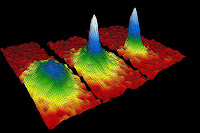### Fast solvers for the quantum Boltzmann equation and the Bose-Einstein condensationBose-Einstein condensate
The interest in the quantum framework of the Boltzmann equation has in­creased dramatically in the recent years. Although the quantum Boltzmann equation (QBE) for a single specie of particles is valid for a gas of fermions as well as for a gas of bosons, blow up of the solution in finite time may occur only in the latter case. In particular this equation has been successfully used for computing non­equilibrium situations where Bose­Einstein condensate occurs.
At very low but finite temperature a large fraction of the atoms would go into the lowest energy quantum state. This phenomenon is now called Bose­-Einstein condensation (see [link]). Experimentally this has been achieved thanks to strong advancements in trapping and cooling techniques for neutral atoms leading to the 2001 Nobel prize in physics by E.A.Cornell, W.Ketterle and C.E.Wiemann ([here] is a movie of BEC in Rb-87).
In the sequel we report some animated movies of numerical simulations in quantum kinetic theory. Simulations have been computed using the fast method developed in  and refers both to the case of a gas of bosons and of a gas of fermions. In particular in the second movie numerical evidence of the Bose-Einstein condensation is given. We refer to [1,2] for the simulations details.

1. Subcritical regime, regular Bose-Einstein stationary state

2. Critical regime, Bose-Einstein condenstaion

3.The case of Fermions, Fermi-Dirac steady state. Same initial data as in 1.

References
1. P.Markowich, L.Pareschi, Fast, conservative and entropic numerical methods for the Bosonic Boltzmann equation, Numerische Math. 99 (2005), pp.509--532.
2. P.Markowich, L.Pareschi, W.Bao, Quantum kinetic theory: modelling and numerics for Bose-Einstein condensation, Chapter 10, Modeling and computational methods for kinetic equations, Series: Modeling and Simulation in Science, Engineering and Technology, Birkhauser (2004), pp.287-320.